# Count Total Number of Cells in a Range – Excel & Google Sheets

This tutorial will demonstrate how to count the total number of cells in a given range. This requires the use of two functions, the ROWS and COLUMNS function.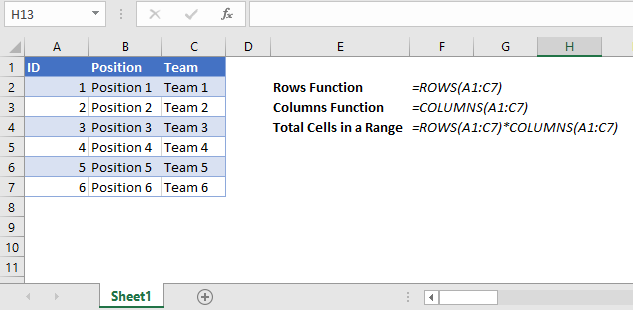## ROWS Function

The ROWS function calculates the total number of rows in a range. Its syntax is:

``=ROWS(Range)``

To illustrate this, see the example below.  The range of cells A1 to A5 cover 5 rows.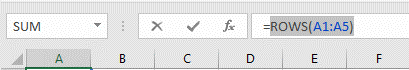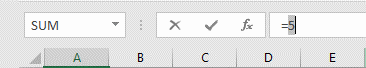Highlight a portion of the function in the formula bar and press F9 to see the calculated value of that formula portion.

## COLUMNS Function

The COLUMNS function is the same as the ROWS function except that it counts the total number of columns in a range. Its syntax is:

``=COLUMNS(Range)``

To illustrate this, see the example below.  The range of cells A1 to D1 cover 4 columns.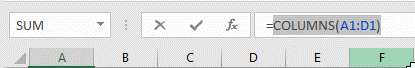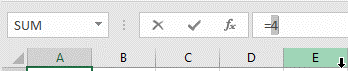## Total Cells in a Range

We can use the ROWS function and the COLUMNS function to find the total number of cells. By multiplying the total number of rows with the total number of columns in the same range, this gives us the total number of cells.

To illustrate this, see the example below.  The range of cells A1:D5 covers 5 rows and 4 columns.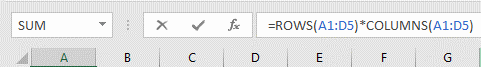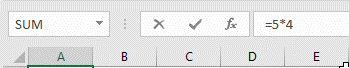Ensure that the range you have inputted in both the ROWS and COLUMNS function is the same.

## Total Cells in a Range for Google Sheets

You can use the same functions on Google Sheets to get to the same result.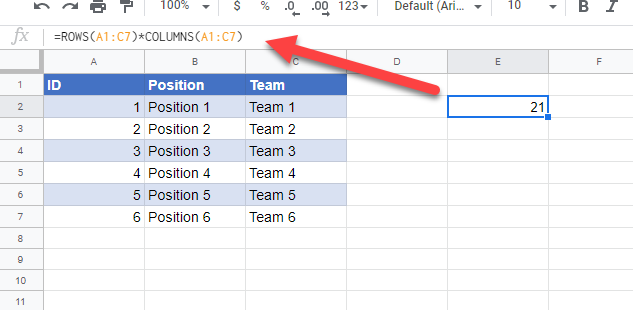### Excel Practice Worksheet

Practice Excel functions and formulas with our 100% free practice worksheets!